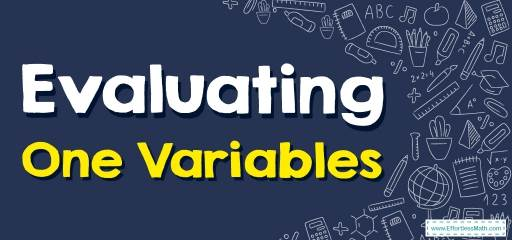# How to Evaluate One Variable? (+FREE Worksheet!)

Some mathematical expressions have one variable. Learn how to evaluate one variable expressions.## Step by step guide to evaluating one variable

• To evaluate one variable expression, find the variable and substitute a number for that variable.
• Perform arithmetic operations.

### Evaluating One Variable – Example 1:

Solve this expression when $$x=2$$,  $$18 \ – \ 2x$$

Solution:

First substitute $$2$$ for $$x$$, then:
$$18 \ − \ 2 x=18 \ − \ 2(2)=18 \ − \ 4=14$$

### Evaluating One Variable – Example 2:

Solve this expression. $$5 \ – \ 2 x ,x=\ – \ 1$$

Solution:

First substitute $$- \ 1$$ for $$x$$, then:
$$5 \ − \ 2 x=5 \ − \ 2 \ (- 1)=5 \ + \ 2=7$$

### Evaluating One Variable – Example 3:

Solve this expression. $$12-2x ,x=-1$$

Solution:

First substitute $$-1$$ for $$x$$, then:
$$12-2x=12-2(-1)=12+2=14$$

### Evaluating One Variable – Example 4:

Solve this expression. $$-8+5x ,x=3$$

Solution:

First substitute $$3$$ for $$x$$, then:
$$-8+5x=-8+5(3)=-8+15=7$$

## Exercises for Evaluating One Variable

### Simplify each algebraic expression.

• $$\color{blue}{9 – x , x = 3}$$
• $$\color{blue}{x + 2, x = 5}$$
• $$\color{blue}{3x + 7, x = 6}$$
• $$\color{blue}{(– 3) + \frac{x}{4} + 2x, x = 16}$$
• $$\color{blue}{(– 2) + \frac{x}{7}, x = 21}$$
• $$\color{blue}{(– \frac{14}{x}) – 9 + 4x, x = 2}$$

• $$\color{blue}{6}$$
• $$\color{blue}{7}$$
• $$\color{blue}{25}$$
• $$\color{blue}{33}$$
• $$\color{blue}{1}$$
• $$\color{blue}{-8}$$

### What people say about "How to Evaluate One Variable? (+FREE Worksheet!) - Effortless Math: We Help Students Learn to LOVE Mathematics"?

No one replied yet.

X
30% OFF

Limited time only!

Save Over 30%

SAVE $5 It was$16.99 now it is \$11.99